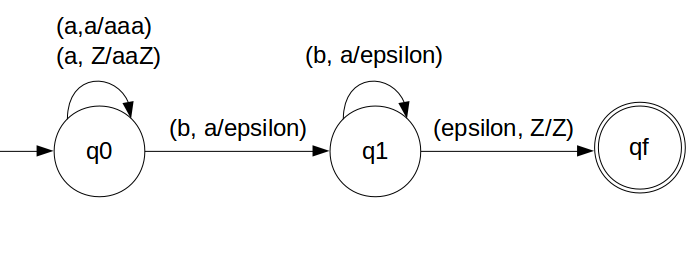DPDA for anb2n n ≥ 1

For every two a's push two a's into STACK cause there are two b's for one 'a'
So by pushing two 'a' we can have 'a' for every 'b'.
That we will achieve by pushing two a's and poping a's for every b
And then pushing c's and poping c's for every d's
So in the end of the strings if nothing is left in the STACK then we can say that language is accepted in the PDA.

We have designed the PDA for the problem:### STACK Transiton Function

```		δ(q0, a, Z) = (q0, aaZ)
δ(q0, a, a) = (q0, aaa)
δ(q0, b, a) = (q1, ε)
δ(q1, b, a) = (q1, ε)

δ(q1, ε, Z) = (qf, Z)
```

Note: qf is Final State

### Explanation

Lets see, how this DPDA is working:
We will take one input string: "aabbbb"
• Scan string from left to right
• First input is 'a' and follow the rule:
• on input 'a' and STACK alphabet Z, push the two 'a's into STACK as : (a,Z/aaZ) and state will be q0
• Second input is 'a' and so follow the rule:
• on input 'a' and STACK alphabet 'a', push the two 'a's into STACK as : (a,a/aaa) and state will be q0
• Now the STACK has "aaaa", So:
• Third input is 'b' and so follow the rule:
• on input 'b' and STACK alphabet 'a', pop one 'a' from STACK as : (b,a/ε) and state will be q1
• Fourth input is 'b' and so follow the rule:
• on input 'b' and STACK alphabet 'a' and state q1, pop one 'a' from STACK as : (b,a/ε) and state will remain q1
• Fifth input is 'b' and so follow the rule:
• on input 'b' and STACK alphabet 'a', pop one 'a' from STACK as : (b,a/ε) and state will be q1
• Sixth input is 'b' and so follow the rule:
• on input 'b' and STACK alphabet 'a' and state q1, pop one 'a' from STACK as : (b,a/ε) and state will remain q1
• We reached end of the string, so follow the rule:
• on input ε and STACK alphabet Z, go to final state(qf) as : (ε, Z/Z)

• The explanation might be looking over descriptive but please be patient, It will all get clear once you start to get along with the explanation.
So we suggest you to keep doing the practice with the steps mentioned in the explnation